• 一、一维数组传参 #include<stdio.h> #include<stdlib.h> #include <stdio.h> void test(int arr[])//ok? yes {} void test(int arr)//ok? yes {} void test(int *arr)//ok? yes {} void ...
一、一维数组传参
#include<stdio.h>
#include<stdlib.h>
#include <stdio.h>
void test(int arr[])//ok? yes
{}
void test(int arr)//ok? yes
{}
void test(int *arr)//ok? yes
{}
void test2(int *arr)//ok? yes
{}
void test2(int **arr)//ok? yes
{}
int main()
{
//int arr;//数组
//int *parr1;//指针数组，元素是指针，可以定义指向
//int(*parr2);//数组指针
//int(*parr3);//数组指针数组
int arr = { 0 };
int *arr2 = { 0 };
test(arr);//看类型是否匹配
test2(arr2);
system("pause");
return 0;
}
传参首先要保证形参和实参的类型要匹配，
在这里笔者重点解释
void test2(int **arr)//ok? yes
{}
int *arr2 = { 0 };
二级指针传参：
arr2是数组名，数组名的类型是 int *，指针数组arr2,的类型就是int **，所以可以用二重指针去接收。
#include <stdio.h>
#include<stdlib.h>
int main() {
int arr[] = { 1, 2, 3 };
int* p = arr;
// 改变之前 p 的地址
printf("%p\n", p);
// q 用来保存改变之前 p 的地址
int* q = p;
// 改变之后 p 的地址
printf("%p\n", p);
printf("%d\n", q);
// 地址改变的差值
printf("%lu\n", p - q);
system("pause");
return 0;
}
*value += sizeof(int);
}

//没错, 如果说一级指针是用于对数据的更新, 那么二级指针就是用于对数据地址的更新
//以此类推, 三级指针就是对数据地址的地址的更新…

展开全文一维数组传参 二维数组传参 一级指针传参
• 一维数组传参 1.在一个数组中查找一个数 #include <stdio.h> #include <stdlib.h> int main(){ int arr = { 9, 5, 2, 7 }; int Tofind = 2; for (int i = 0; i < 4;i++){ if (arr[i] != To...
一维数组传参
1.在一个数组中查找一个数
#include <stdio.h>
#include <stdlib.h>

int main(){
int arr = { 9, 5, 2, 7 };
int Tofind = 2;
for (int i = 0; i < 4;i++){
if (arr[i] != Tofind){
continue;
}
printf("%d\n",i);
}
system("pause");
return 0;
}

运行结果: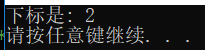拓展
#include <stdio.h>
#include <stdlib.h>

int Func(int arr[],int size){
int Tofind = 2;
for (int i = 0; i < 4; i++){
if (arr[i] != Tofind){
continue;
}
return i;
}
}

int main(){
int arr = { 9, 5, 2, 7 };

printf ("%d\n",Func(arr, 4));
system("pause");
return 0;
}

运行结果: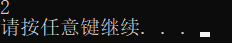二维数组传参的三种形式
1.打印一个二维数组
#include <stdio.h>
#include <stdlib.h>

int main(){
//长度为3,每个元素的长度是4
int arr = { 1, 2, 3, 4, 5, 6, 7, 8, 9, 10, 11, 12 };
for (int row = 0; row < 3;row++){
for (int col = 0; col < 4; col++){
printf(" %d ", arr[row][col]);
}
printf("\n");
}

system("pause");
return 0;
}

运行结果: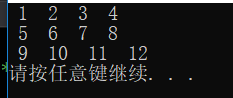法1.
#include <stdio.h>
#include <stdlib.h>

void Printarr(int arr[]){
for (int row = 0; row < 3; row++){
for (int col = 0; col < 4; col++){
printf(" %d ", arr[row][col]);
}
printf("\n");
}
}

int main(){
//长度为3,每个元素的长度是4
int arr = { 1, 2, 3, 4, 5, 6, 7, 8, 9, 10, 11, 12 };
Printarr(arr);

system("pause");
return 0;
}

运行结果:法2.
#include <stdio.h>
#include <stdlib.h>

void Printarr(int arr){
for (int row = 0; row < 3; row++){
for (int col = 0; col < 4; col++){
printf(" %d ", arr[row][col]);
}
printf("\n");
}
}

int main(){
//长度为3,每个元素的长度是4
int arr = { 1, 2, 3, 4, 5, 6, 7, 8, 9, 10, 11, 12 };
Printarr(arr);

system("pause");
return 0;
}

法3.
#include <stdio.h>
#include <stdlib.h>

//数组函数传参的时候会隐式转成指针,这个指针就是指向首元素的指针
//当前参数是一个二维数组,首元素是一个int
//首元素的指针就是int (*) 数组指针
void Printarr(int (*arr)){
for (int row = 0; row < 3; row++){
for (int col = 0; col < 4; col++){
printf(" %d ", arr[row][col]);
}
printf("\n");
}
}

int main(){
//长度为3,每个元素的长度是4
int arr = { 1, 2, 3, 4, 5, 6, 7, 8, 9, 10, 11, 12 };
Printarr(arr);

system("pause");
return 0;
}

运行结果:int arr //属于普通数组 int* arr2 //指针数组 int(*p) //数组指针 int(*p) //长度为10个元素的指针数组,里面的每一个元素都是一个数组指针,这个数组指针指向长度为5个元素的int数组
一级指针传参
#include <stdio.h>
#include <stdlib.h>

void Print(int* arr, int size){
int i = 0;
for (i = 0; i < size;i++){
printf(" %d ",*(arr+i));
}
printf("\n");
}
int main(){
int arr = { 0, 1, 2, 3, 4, 5, 6, 7, 8, 9 };
int* p = arr;
int size = sizeof(arr) / sizeof(arr);
//把一级指针p传给函数
Print(p,10);
system("pause");
return 0;

}

运行结果: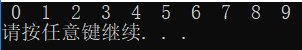二级指针传参
#include <stdio.h>
#include <stdio.h>

void test(int** ptr){

printf("%d\n",**ptr);
}
int main(){

int n = 10;
int* p = &n;
int** pp= &p;

//test(pp);
test(&p);
system("pause");
return 0;
}

运行结果: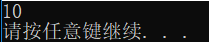展开全文• 指针汇总字符指针指针数组数组指针二级指针传参函数指针 字符指针 #include "stdio.h" int main(){ char* ptr = "hello bit"; return 0; } 这里并不是把"hello bit"整个字符串传给指针ptr，指针ptr只是只想字符串...


指针汇总
字符指针指针数组数组指针二级指针传参函数指针函数指针数组指向函数指针数组的指针回调函数

字符指针
#include "stdio.h"
int main(){
char* ptr = "hello bit";
return 0;
}

这里并不是把"hello bit"整个字符串传给指针ptr，指针ptr只是只想字符串首字母"h"的地址
#include "stdio.h"
int main(){
char str1[] = "hello";
char str2[] = "hello";
char *ptr1 = "hello";
char *ptr2 = "hello";
return 0;
}

这里str1和str2都是创建新的数组空间存放字符串"hello"，而ptr1和ptr2只是两个指针，其只想一片存放"hello"的地址，ptr1和ptr2相等，而str1和str2不等。且char *ptr="hello"这样ptr表示常量字符串，是不能修改的，可以写成const char *ptr = “hello”
指针数组
指针数组是一个元素为指针的数组
int* arr1;

名字arr先与[]结合表示这是一个数组，里面存放int类型的元素。 去除数组名arr，剩下int 就是数组类型
数组指针
int (*p)

因为[]优先级高于*，所以使用(*p)表示p是一个指针；将(*p)去掉后剩余int 为p指向的类型，是存放10个元素的数组，每个元素类型为int
数组指针使用场景
#include "stdio.h"
void my_print(int (*ptr), int a, int b){
//    传过来的是一个数组的地址&a，a是存有两个元素的数组的数组名，故用指向数组的指针接收
for (int i = 0; i < a; ++i) {
for (int j = 0; j < b; ++j) {
//            printf("%d ",ptr[i][j]);
printf("%d ",*((*(ptr+i))+j));   //  (ptr+i)得到第i行数组的首地址，*(ptr+i)对数组地址解引用得到数组，相当于得到一维数组数组名，*(ptr+i)其是数组首元素地址
}
}
}

int main(){
//    在非siezeof情况下，二维数组数组名arr表示第一行数组的地址，若第一行存放a={0},则arr = &a;
//    因此 *arr得到第一行数组，即*arr相当于第一行数组名a，其存放的与a一样（无sizeof等两种特殊情况），是第一行数组首元素a的地址；
//    *arr 与 arr等价，arr = *(arr+0)
int arr = {{1,2},{3,4},{5,6}};
my_print(arr,3,2);  //这里不是sizeof情况，arr单独出现，表示的应该是首行数组的地址（一个数组的地址，相当于&a）
return 0;
}

二级指针传参
#include "stdio.h"
void test(char* *P){  //p是一个指针，其指向一个地址（指针），该指针指向的元素是char

}

int main(){
char c = 'b';
char *pc = &c;
char* *pcc = &pc;
char* arr;
test(&pc);    //pc是一个指针，其指向char，传地址过去符合
test(pcc);    //pcc是一个指针，其存放的是一个地址，该地址指向char，因此pcc丢进去就传进去了一个指向char类型元素地址的地址
test(arr);    //arr是一个数组名，其存放指向第一个元素的地址，第一个元素为char * ；相当于传进去了char * 的地址，用char* *p接受正合适
return 0;
}

函数指针
int (*p) (int, int)

(*p)表示指针；(int,int)与(*p)结合表示该指针为函数指针，(int,int)其本身表示指向的函数的两个参数类型；将(*p)和(int int)去掉后剩余的int表示指向函数的返回类型
#include "stdio.h"
void test(){
printf("hehe\n");
}
int main(){
printf("%p\n",test);
printf("%p\n",&test);
return 0;
}

对于函数名来说，这两种一样，都是函数地址
同样，对于(*p)中的*也只是个摆设，为了容易理解而写而已；完全可以(*p)(a,b) -> p(a,b)

函数指针数组
int (*ptr)()
//函数指针数组是数组，所以名称应该与[]先结合

首先ptr与先结合，说明ptr这个名称表示数组，有10个元素；把ptr去掉可知剩余的int (*)()是数组每个元素的类型，其为函数指针类型，可知这是函数指针数组（其他情况一样分析）
指向函数指针数组的指针
一个指针指向一个数组，数组元素都是函数指针
//一个函数指针
void (*ptr)(char *)

//一个指针数组
void (*ptrr)(char *)

//*首先与ptrrr结合(*ptrrr)表明ptrrr是个指针；紧接着(*ptrrr)与结合(*ptrrr)表明指针指向数组；将(*ptrrr)这部分去掉，剩余的void (*)(char *)表示数组元素类型为函数指针类型
void (*((*ptrrr)))(char *)

回调函数
回调函数就是一个通过函数指针调用的函数。如果把函数的指针（地址）作为参数传给另一个函数，当这个指针被用来调用其指向函数时，这个被指向的函数叫回调函数。
展开全文• 二级指针 void GetMemory（char** p,intnum） {  *p = (char*)malloc(num*sizeof(char));  returnp； } int main() {  char*str= NULL;  GetMemory（&str，10）;  strcpy(str,”hello”);  fr


根据《C语言深度解析》

二级指针

void GetMemory（char** p,intnum） {     *p = (char*)malloc(num*sizeof(char));      returnp； } int main() {      char*str= NULL;      GetMemory（&str，10）;      strcpy(str,”hello”);      free（str） ；      return0; }

注意main函数传参是传的&str，传入到函数的是str的地址，是一个值，不是str的拷贝，而

*p就相当于*(&str)也就是str本身，所以经过这个函数，str的值被改变了，那么*str指向的就是新申请的堆内存的地址空间。

函数指针：

#include <stdio.h>

#include <string.h>
char* fun(char* p1,char* p2)
{
inti = 0;
i = strcmp(p1,p2);
if (0 == i)
{
returnp1;
}
else
{
returnp2;
}
}
int    main()
{
char* (*pf)(char* p1,char* p2);
pf = &fun;
(*pf)(“aa”,”bb”);
return0;
}

pf不是一个函数名，而是一个指针，这个指针指向的是返回值为char*的函数，使用的时候，需要通过

*pf来使用。

注意差别：

A)，char* (*fun1)(char* p1,char* p2);//fun1函数指针

B)，char* *fun2(char* p1,char* p2);//fun2位函数名，返回值为二级指针

C)，char* fun3(char* p1,char* p2);//fun3也是函数名，返回的是一级指针

理解*(int *)&p:

void Function() {     printf(“Call Function!\n”); } int main() {     void (*p)();     *(int*)&p=(int)Function;     (*p)();     return 0; }

这是在干什么？*(int*)&p=(int)Function;表示什么意思？

先看void (*p)();

意思是定义了一个指针，指向一个返回值和参数都为void 类型的函数。

那么&p就是取这个指针变量本身所在的地址，

(int*)&p 表示将地址强制转换成指向 int 类型数据的指针

(int )Function表示将Function()函数的入口地址转化为int型数据

那么*(int*)&p=(int)Function;这句话的意思就是将Function()函数的地址赋给指针变量p，

(*p)();表示调用这个函数。

使用函数指针的好处在于， 可以将实现同一功能的多个模块统一起来标识， 这样一来更

容易后期的维护，系统结构更加清晰。或者归纳为：便于分层设计、利于系统抽象、降低耦

合度以及使接口与实现分开。

不成魔不能活：

(*(void(*) ())0)();查看这个例子

按照结合的优先级:

void(*)()是一个函数指针，指向的是一个参数和返回值都为void类型的函数，

void(*)()0，表示将地址0强制转换为函数指针类型，0就是一个地址，也就是说有一个函数存在以0开头的一段地址中。

(*(void(*) ())0)()；意思就是调用存放在0地址处的返回值和参数都为void类型的函数。

那么下面这句话也好理解：

(*(char**(*)(char**,char**))0)( char**,char**);

函数指针数组：存放指向函数的指针的数组

char* (*pf)(char* p);

那么这里面的pf不是指针，而是一个数组名，如果想要数组，那么就需要在整体加上*，也就是

char* (*(*pf))(char* p);这个称为
函数指针数组的指针

经典指定程序的运行位置的方法，函数指针法

typedef void (lpFuntion*)(); lpfunction lpReset = (lpfunction)0xff0000f0; lpReset();


展开全文• 二维数组作为实参，二级指针作为形参 part1 报错：说我给二级指针传了一个数组指针去，数组指针（指向数组的指针）， part2 给二级指针传一个数组指针就可以解决了。 part3(总结) 把指针数组和数组指针好好区别...
• 二级指针 void GetMemory（char** p,intnum） {  *p = (char*)malloc(num*sizeof(char));  returnp； } int main() {  char*str= NULL;  GetMemory（&str，10）;  strcpy(s函数 c语言深度解析
• 给定一批整数，以0作为结束标志且不作为节点，将其建成一个先进先出的链表。 #include #include typedef struct node { int date; struct node *next; }node; void Creatlink(struct node **... struct node *
• 一维数组传参 void test(int arr[]) {} void test(int arr) {} void test(int *arr) {} void test2(int *arr) {} void test2(int **arr) {} int main(){ int arr = {0}; int *arr2 = {0}; .....
• * malloc不应该是返回一个指向这块内存地址的指针，假设这个指针是p，那么malloc那一句应该等价于*L=*p; * 所以说应该是让L指向这块内存，因而L存储的应该是分配的内存地址。 * 怎么结果是使L1指向这个地址了。
• malloc函数返回一个指向内存地址的指针，这个指针值赋值给L指针，因为L存储的是L1自身的存储地址，因此这个操作是不是应该理解为将L1自身的存储地址设为了这块分配的内存地址呢？[/color][/b][/size]
• 3、如果使用二级指针传递，pp作为形参，会产生指针的拷贝pp_cpy，此时pp和pp_cpy都是指向p，所分配的内存的地址自然也就可以传递给*pp（即p）啦！ 以上三点仅是说明str并没有分配到内存，是NULL；编译出错。 ...
• 建立二叉树时，要递归的修改指针的值，可采用的方法有：二级指针、引用（C++）、返回值。 具体使用方法如上示。注意函数的声明方法和调用方法。 ★ 引用和取地址的区别：函数形参表里的&是引用，函数实参表里的&是取...null struct c
• 一维数组的传参/一级指针传参/普通指针 在理解指针的基础上，一维数组的指针传递很简单，我们知道数组的数组名就是这个数组首元素的地址，所以将数组名传入函数就可以了 // 一维数组的传递0.cpp # include <...字符串 c++ c语言 数据结构
• 二级指针传参 一、数组参数、指针参数 在写代码的时候难免要把【数组】或者【指针】传给函数，那函数的参数该如何设计呢？ 1. 一维数组传参 #include <stdio.h> void test(int arr[]) {} void test(int ...c语言
• 级指针传参 传一维数组 #include<stdio.h> #include<stdlib.h> int point(int* arr) { for (int i = 0; i <= 3; ++i) { printf("%d\t", arr[i]); } } int main() { int arr[] = { 1, 2, 3, ......